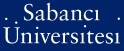# Bari-Markus property for Riesz projections of 1D periodic Dirac operators

Djakov, Plamen Borissov and Mityagin, Boris Samuel (2010) Bari-Markus property for Riesz projections of 1D periodic Dirac operators. Mathematische Nachrichten, 283 (3). pp. 443-462. ISSN 0025-584X

This is the latest version of this item.Preview
PDF (This is a RoMEO green publisher -- author can archive post-print (ie final draft post-refereeing)) - Requires a PDF viewer such as GSview, Xpdf or Adobe Acrobat Reader
456Kb

Official URL: http://dx.doi.org/10.1002/mana.200910003

## Abstract

The Dirac operators Ly = i ((1)(0) (0)(-1))dy/dx + v(x)y, y = ((y1)(y2)), x is an element of[0, pi], with L-2-potentials v(x) = ((0)(Q(x)) (P(x))(0)), P, Q is an element of L-2([0, pi]), considered on [0, pi] with periodic, antiperiodic or Dinchlet boundary conditions (bc), have discrete spectra, and the Riesz projections, S-N = 1/2 pi iota integral(vertical bar z vertical bar=N - 1/2) (z - L-bc)(-1) dz. p(n) = 1/2 pi iota integral(vertical bar z-n vertical bar=1/2) (z - L-bc)(-1) dz are well-defined for vertical bar n vertical bar >= N if N is sufficiently large. It is proved that Sigma(vertical bar n vertical bar>N) parallel to P-n - P-n(0)parallel to(2) < infinity, where P-n(0), n is an element of Z, are the Riesz projections of the free operator. Then, by the Ban Markus criterion, the spectral Riesz decompositions f = SN + Sigma(vertical bar n vertical bar>N) P(n)f, for all f is an element of L-2 converge unconditionally in L-2. (C) 2010 WILEY-VCH Verlag GmbH & Co KGaA, Weinhom

Item Type: Article 1D periodic Dirac operator, Riesz projections, spectral decomposition Q Science > QA Mathematics > QA299.6-433 Analysis 13824 Plamen Borissov Djakov 12 Mar 2010 11:11 22 Jul 2019 14:36

### Available Versions of this Item

Repository Staff Only: item control page